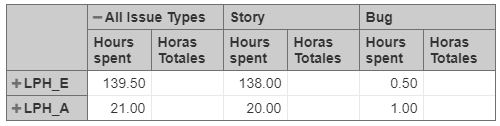# All Issye Type Value

Hi Community,

I want to create the following calculate value:

Issue Type . Hours Spent / All Issue Types . Hours Spent

138 / 139.50 = 0.989247311827957 for Story and
0.50/ 139.50 = 0.003584229390681 for BugHello Humberto_Ramirez_Ant,

Yes , do following steps to achieve above scenario,

1. Create a tuple for total and each issue types as follow,

Calculate hours spent by issue type: Name it as your wish, ex assume the name is A
([Issue Type].CurrentMember,
[Measures].[Hours spent])

Total of all issue types: Name it as your wish, ex assume the name is B
([Issue Type].DefaultMember,
[Measures].[Hours spent])

Then create calculated member like below

A/B